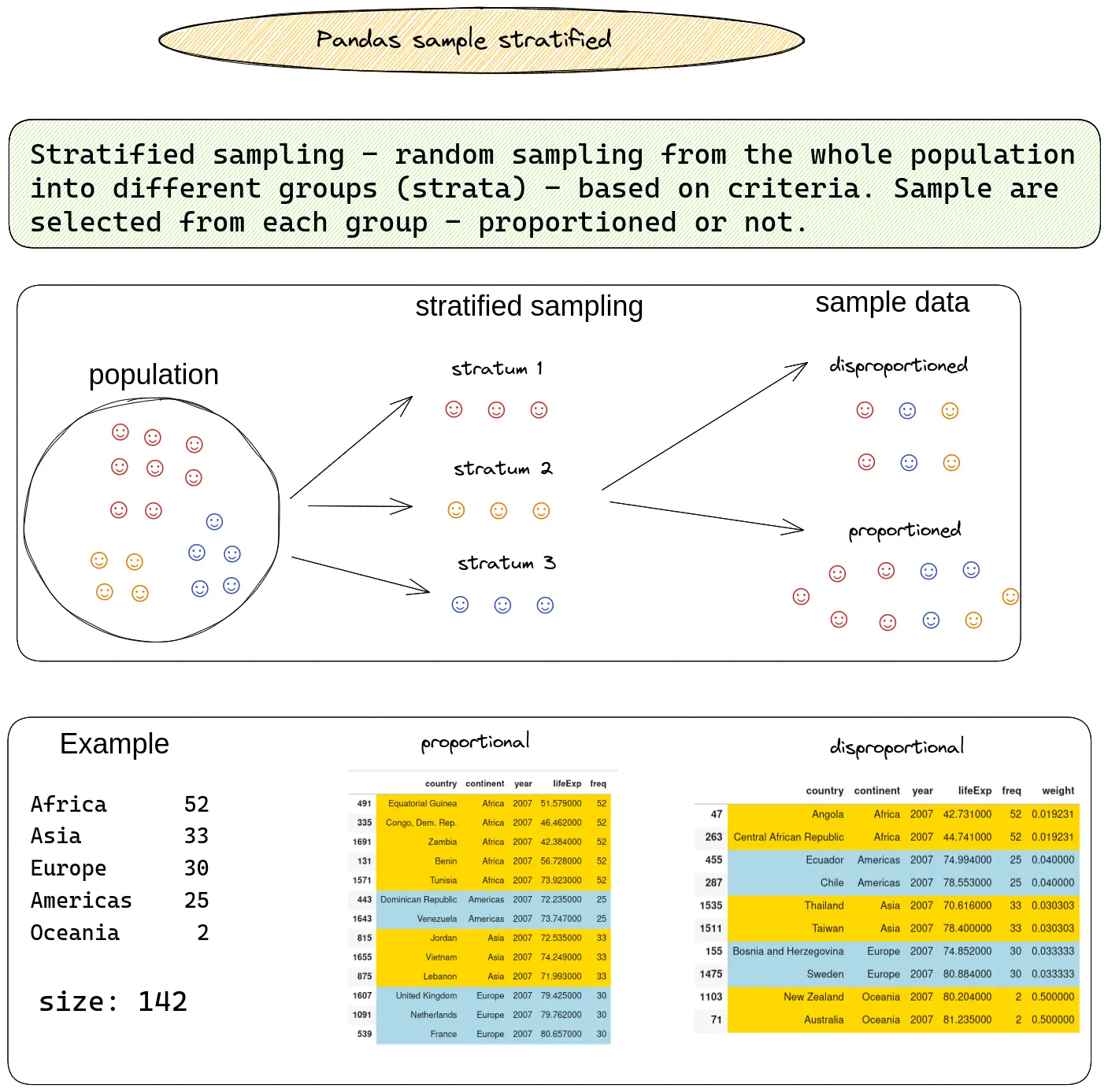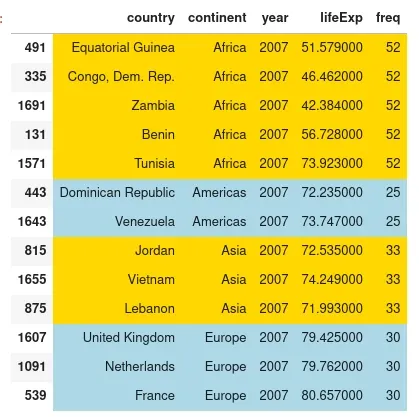In this quick tutorial, we're going to discuss stratified sampling in Pandas and Python.

The following syntax can be used to sample stratified in Pandas:

(1) stratified sampling - disproportionated

``````(df
.groupby('continent', group_keys=False)
.apply(lambda x: x.sample(2))
)
``````

(2) stratified sampling - proportional

``````(df
.groupby('continent', group_keys=False)
.apply(lambda x: x.sample(frac=0.1))
)
``````

The image below illustrates the technique called stratified sampling:Next, you'll see the steps to do stratified sampling in practice.

## Setup

First, let's create a sample DataFrame:

``````import plotly.express as px
df = px.data.gapminder().query("year == 2007")
cols = df.columns[:4]
df = df[cols]
``````

data has the following shape:

``````(142, 5)
``````

First rows of this DataFrame

country continent year lifeExp freq
11 Afghanistan Asia 2007 43.828 33
23 Albania Europe 2007 76.423 30
35 Algeria Africa 2007 72.301 52
47 Angola Africa 2007 42.731 52
59 Argentina Americas 2007 75.320 25

## Separate population into strata

The whole population of this dataset is 142 countries.

Below you can find the proportions per each stratum:

``````df[col_name].value_counts()
``````

result is:

``````Africa  	52
Asia    	33
Europe  	30
Americas	25
Oceania  	2
Name: continent, dtype: int64
``````

## Find the sample size

Next we need to decide on what should be the size of the sample. There are different strategies on this.

These are the options available in Pandas `sample()` method:

• `n` - number of items to return. We get N random items
• `frac` - fraction of items to return
• `weights` - probability weighting

So we can select the size of:

• total sample as percentage on the whole population
• each group
• disproportionated
• proportionated

## Disproportional stratified sampling

In this approach the size of each sample group is not proportional to the entire population.

We will get equal number of items for each group:

``````(df
.groupby('continent', group_keys=False)
.apply(lambda x: x.sample(2))
)
``````

The result is 2 sized stratum - no matter the size of each group:

country continent year lifeExp freq
911 Libya Africa 2007 73.952 52
899 Liberia Africa 2007 45.678 52
443 Dominican Republic Americas 2007 72.235 25
791 Jamaica Americas 2007 72.567 25
1679 Yemen, Rep. Asia 2007 62.698 33
1319 Saudi Arabia Asia 2007 72.777 33
779 Italy Europe 2007 80.546 30
1607 United Kingdom Europe 2007 79.425 30
1103 New Zealand Oceania 2007 80.204 2
71 Australia Oceania 2007 81.235 2

## Proportional stratified sampling

Taking random sampling from stratified groups which is proportional to the population.

We can do proportional stratified sampling in Pandas by sampling with parameter `x.sample(frac=0.1)`:

``````(df
.groupby('continent', group_keys=False)
.apply(lambda x: x.sample(frac=0.1))
)
``````

This will give us countries proportioned to the initial population:

country continent year lifeExp freq
491 Equatorial Guinea Africa 2007 51.579 52
335 Congo, Dem. Rep. Africa 2007 46.462 52
1691 Zambia Africa 2007 42.384 52
131 Benin Africa 2007 56.728 52
1571 Tunisia Africa 2007 73.923 52
443 Dominican Republic Americas 2007 72.235 25
1643 Venezuela Americas 2007 73.747 25
815 Jordan Asia 2007 72.535 33
1655 Vietnam Asia 2007 74.249 33
875 Lebanon Asia 2007 71.993 33
1607 United Kingdom Europe 2007 79.425 30
1091 Netherlands Europe 2007 79.762 30
539 France Europe 2007 80.657 30

Africa is the most represented continent while Oceania is missing from this sample.

### color row based on column value

If you like to learn how to style each group into different color check: color Pandas DataFrame based on value

``````def format_color_groups(df):
colors = ['gold', 'lightblue']
x = df.copy()
factors = list(x['continent'].unique())
i = 0
for factor in factors:
style = f'background-color: {colors[i]}'
x.loc[x['continent'] == factor, :] = style
i = not i
return x

d1.style.apply(format_color_groups, axis=None)
``````

The result is:## group by sample weights in Pandas

We can use weights to get proportional sampling. First we need to calculate the weights of each stratum:

### Calc weights - groupby + .transform('count')

``````df['weight'] = (df
.groupby('continent')
.country
.transform('count')
)
``````

as a result we have Pandas series which contains the weight for each row:

``````11  	33
23  	30
35  	52
47  	52
59  	25
..
1655	33
1667	33
1679	33
1691	52
1703	52
Name: country, Length: 142, dtype: int64
``````

### Calc weights - equal representation

To get equal representation of each group we can calculate the weights:

``````df['weight'] = 1./(df
.groupby('continent')
.country
.transform('count')
)
``````

The get disproportional rate:

``````11  	0.030303
23  	0.033333
35  	0.019231
47  	0.019231
59  	0.040000
...
1655	0.030303
1667	0.030303
1679	0.030303
1691	0.019231
1703	0.019231
Name: weight, Length: 142, dtype: float64
``````

### Calc weights - map and value_counts

We can achieve the same result by `.map` and `.value_counts()`

``````df['weight'] = (df
.continent
.map(df['continent'].value_counts())
)
``````

### sampling with weights

To do sampling with respect to distribution of a value in a given column we can use the calculate frequency for parameter `weights`:

``````df.sample(n=10, weights = df['weight'])
``````

Result is weighted sampling:

country continent year lifeExp freq weight
851 Korea, Rep. Asia 2007 78.623 33 33
491 Equatorial Guinea Africa 2007 51.579 52 52
1043 Mozambique Africa 2007 42.082 52 52
1175 Pakistan Asia 2007 65.483 33 33
1223 Philippines Asia 2007 71.688 33 33
1667 West Bank and Gaza Asia 2007 73.422 33 33
1031 Morocco Africa 2007 71.164 52 52
1139 Nigeria Africa 2007 46.859 52 52
1559 Trinidad and Tobago Americas 2007 69.819 25 25
635 Guinea-Bissau Africa 2007 46.388 52 52

## Conclusion

In this article, we took a closer look at stratified sampling in Pandas and how to apply it in practice. We covered the two main approaches in stratified sampling - disproportionated and proportionated.

Finally we explain how to use weights to sample and groupby in Pandas DataFrame.